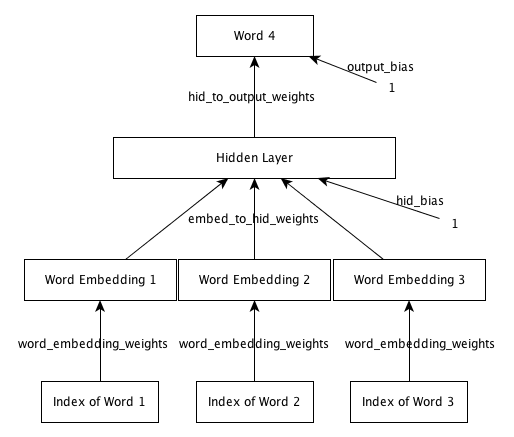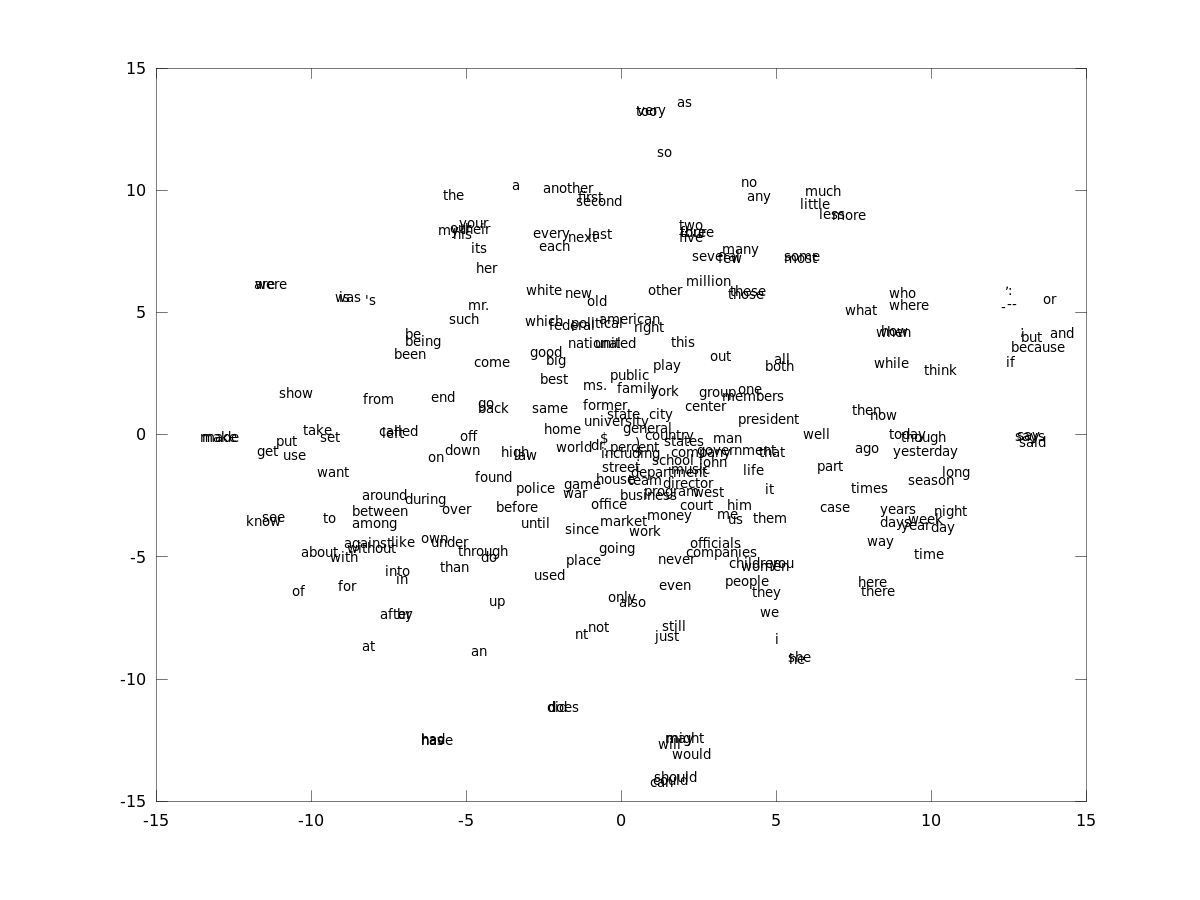CSC 321: Assignment 1

CSC 321: Assignment 1 - Learning distributed word representations

TA: Nitish Srivastava, Jian Yao (csc321a@cs.toronto.edu)

In this assignment we will make neural networks learn about words. One way to do this is to train a network that takes a sequence of words as input and learns to predict the word that comes next.

Code

Slides from tutorial [pdf].

Getting started

Look at the file raw_sentences.txt. It contains the sentences that we will be using for this assignment. Sequences of 4 adjacent words (called 4-grams) were extracted from them. These sentences are fairly simple ones and cover a vocabulary of only 250 words.

Dataset

The data set consists of 4-grams extracted from raw_sentences.txt. All the words involved come from a small vocabulary of 250 words.

Open a matlab terminal and load data.mat. It should contain the training, validation and test sets, along with the 250-word vocabulary. 'data.trainData' is a 4 x 372,500 matrix. This means there are 372,500 training cases and 4 words per training case. Each entry is an integer that is the index of a word in the vocabulary. So each column represents a sequence of 4 words. 'data.validData' and 'data.testData' are also similar. They contain 46,500 4-grams each.

Training the model

train.m trains a neural net language model. It loads the data and trains a neural net to predict the fourth word given the previous three words of a 4-gram. It takes two arguments-
• d: The number of dimensions in the distributed representation.
• num_hid: The number of hidden units
For example, execute the following
model = train(8, 64);
As the model trains, the script prints out some numbers that tell you how well the training is going. It shows -
• The cross entropy on the last 100 mini-batches of the training set. This is shown after every 100 mini-batches.
• The cross entropy on the entire validation set every 1000 mini-batches of training.
At the end of training, the script shows the cross entropies on the training, validation and test sets. It will output a 'model' (a struct containing weights and biases of the network and the vocabulary).

Network ArchitectureThe network consists of an input layer, embedding layer, hidden layer and output layer. The input layer consists of three word indices. The same 'word_embedding_weights' are used to map each index to a distributed feature representation. These mapped features constitute the embedding layer. This layer is connected to the hidden layer, which in turn is connected to the output layer. The output layer is a softmax over the 250 words.

Some useful functions

These functions can be used for analyzing the model after the training is done.
• tsne_plot.m: This method will create a 2 dimensional embedding of the distributed representation space using t-SNE. From the learned model, you can create pictures that look like this -• display_nearest_words.m : This method will display the words closest to a given word in the word representation space.
• word_distance.m : This method will compute the distance between two given words.
• predict_next_word.m : This method will produce some predictions for the next word given 3 previous words.
Take a look at the documentation inside these functions to see how to use them.

Fun things to try

• Choose some words from the vocabulary and make a list. Find the words that the model thinks are close to words in this list (for example, find the words closest to 'companies', 'president', 'day', 'could', etc). Do the outputs make sense ?
• Pick three words from the vocabulary that go well together (for example, 'government of united', 'city of new', 'life in the', 'he is the' etc). Use the model to predict the next word. Does the model give sensible predictions?
• Which words would you expect to be closer together than others ? For example, 'he' should be closer to 'she' than to 'federal', or 'companies' should be closer to 'business' than 'political'. Find the distances using the model.Do the distances that the model predicts make sense ?

What you have to do

You must train the model four times, trying all possible combinations of d=8,d=32 and num_hid=64,num_hid=256. You must record the final cross entropy error on the training, validation, and test sets for each of these runs. You must also record the number of epochs at which the training stops. These four quantities are written into the model struct.

Select the best configuration that you ran. The function word_distance has been provided for you so that you can compute the distance between the learned representations of two words. The word_distance function takes two strings and the model as arguments. For example, if you wanted to compute the distance between the words "and" and "but" you would do the following (after training the model of course).

word_distance('and', 'but', model)

The word_distance function simply takes the feature vector corresponding to each word and computes the L2 norm of the difference vector. Because of this, you can only meaningfully compare the relative distances between two pairs of words and discover things like "the word 'and' is closer to the word 'but' than it is to the word 'or' in the learned embedding." If you are especially enterprising, you can compare the distance between two words to the average distance to each of those words. Remember that if you want to enter a string that contains the single quote character in matlab you must escape it with another single quote. So the string apostrophe s, which is in the vocabulary, would have to be entered as '''s' in matlab.

Compute the distances between a few words and look for patterns. See if you can discover a few interesting things about the learned word embedding by looking at the distances between various pairs of words. What words would you expect to be close together? Are they? Think about what factors contribute to words being given nearby feature vectors.

What you have to submit

You should hand in, along with the results you were instructed to record above, some clear, well-written, and concise prose providing a brief commentary on the results. At most two pages will be graded, so anything beyond two pages will be ignored during marking.

Here are a few suggestions on what you should comment on, but they are certainly not exhaustive or meant to limit your discussion. You will be expected to offer some insights beyond what is mentioned below. The questions below are merely to help guide your thinking, please make your analysis go beyond them. You should give your reasoning about why the d and num_hid you found to be the best actually is the best and how you defined "best". Comment a bit on everything you were asked to record and also what seemed to be happening during training. Explain all your observations. What did you discover about the learned word embedding? Why did these properties of the embedding arise? What things can cause two words to be placed near each other?